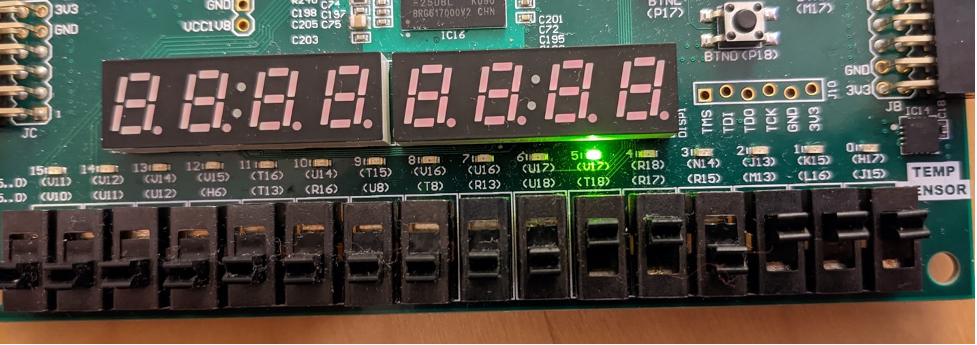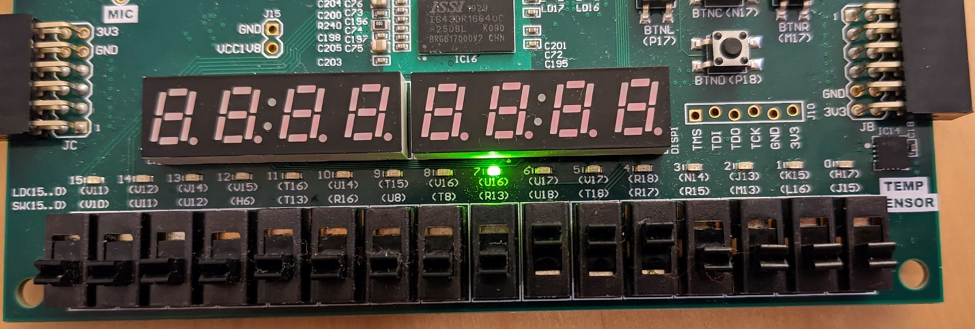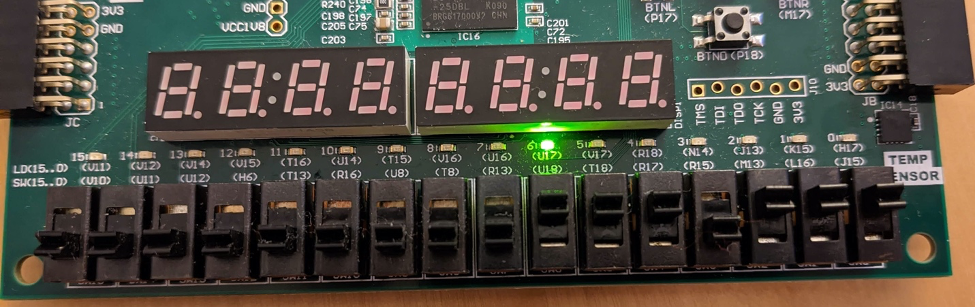# Objective

The objective of this post is to understand how to model a 2-bit comparator and a 4-bit comparator in Verilog. Firstly, a 2-bit comparator is implemented based on the logic expressions from the truth table of each output. Next, likewise, it generates a 4-bit comparator by instantiating two models of the 2-bit comparators and some extra logic gates. Also, the actual results of each output corresponding to the inputs will be observed on the Nexys board based on the own testbench file.

# Environment, Tool, and Board

• Ubuntu 16.04
• Nexys A7 100T (Family: Artix-7, Package: csg324, Speed: -1L)
• Part: xc7a100ticsg324-1L)

# A 2-bit comparator

The truth table of a 2-bit comparator can be represented by the table shown below. Each input (a1, a0, b1, b0) can contain 1 bit of data, and each data will be going into the comparator to compare the size of the data. In the table, the output G stands for “Greater” which means a1a0>b1b0. Likewise, the output E stands for “Equal” which means a1a0=b1b0, and the output L stands for “Lower” which means a1a0<b1b0.

a1a0b1b0GEL
0000010
0001001
0010001
0011001
0100100
0101010
0110001
0111001
1000100
1001100
1010010
1011001
1100100
1101100
1110100
1111010

It is possible to extract the logic expressions of each output from the table. However, in order to minimize the use of gates, the Karnaugh map (K-map) can be useful.

## Logic Expression for output G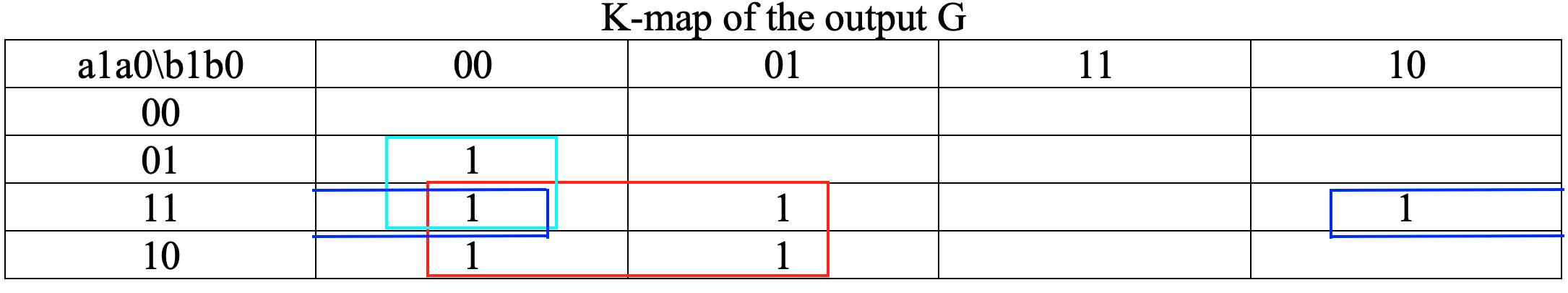G (a1a0>b1b0) = a1b1′ + a0b1′ b0′ + a1a0b0′

Simplifying to reduce the number of the gates by using XNOR gate,

G = a1b1′ + (a1⨀b1)a0b0′

## Logic Expression for output E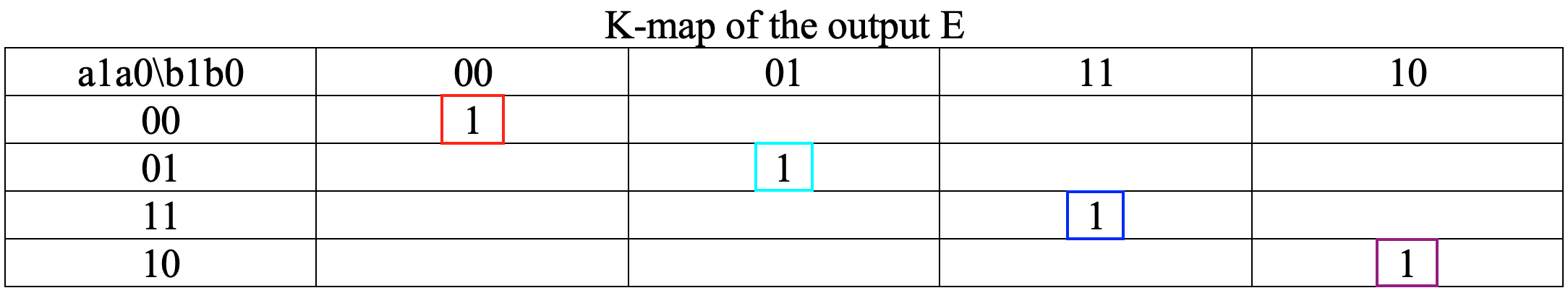E (a1a0=b1b0) = a1’a0’b1’b0′ + a1’a0b1’b0 + a1a0b1b0 + a1a0′ b1b0′

Simplifying to reduce the number of the gates by using XNOR gate,

E = (a1⨀b1)(a0⨀b0)

## Logic Expression for output L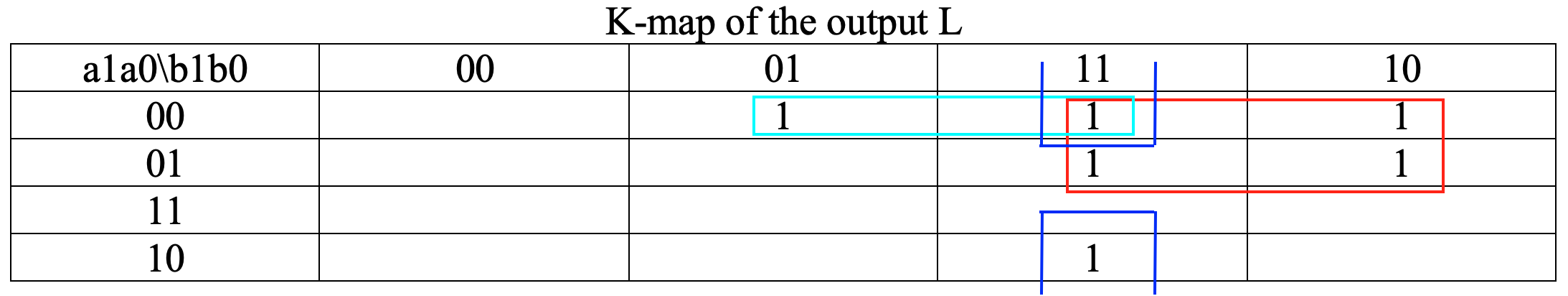L (a1a0<b1b0) = a1’b1 + a1’a0’b0 + a0’b1b0

Simplifying to reduce the number of the gates by using XNOR gate,

L = a1’b1 + (a1⨀b1)a0’b0

# A 4-bit comparator in Verilog

Comparing to the truth table of a 2-bit comparator, a 4-bit comparator will be used 4-bit in input A and 4-bit in input B. Therefore, the truth table of the 4-bit comparator is the following table below.

a3b3a2b2a1b1a0b0GEL
a3 > b3xxx100
a3 < b3xxx001
a3 = b3a2 > b2xx100
a3 = b3a2 < b2xx001
a3 = b3a2 = b2a1 > b1x100
a3 = b3a2 = b2a1 < b1x001
a3 = b3a2 = b2a1 = b1a0 > b0100
a3 = b3a2 = b2a1 = b1a0 < b0001
a3 = b3a2 = b2a1 = b1a0 = b0010

From the table above, the logic expressions of each output are:

G = a3b3′ + (a3⨀b3)a2b2′ + (a3⨀b3)(a2⨀b2)a1b1′ + (a3⨀b3)(a2⨀a2)(a1⨀b1)a0b0′

E=(a3⨀b3)(a2⨀a2)(a1⨀b1)(a0⨀b0)

L = a3’b3 + (a3⨀b3)a2’b2 + (a3⨀b3)(a2⨀b2)a1’b1 + (a3⨀b3)(a2⨀a2)(a1⨀b1)a0’b0

By combining all knowledge learned so far, the source code of the 4-bit comparator in Verilog is:

Then, it can generate a schematic of the 4-bit comparator when clicking “Open Elaborated Design” in Flow Navigator on the left side.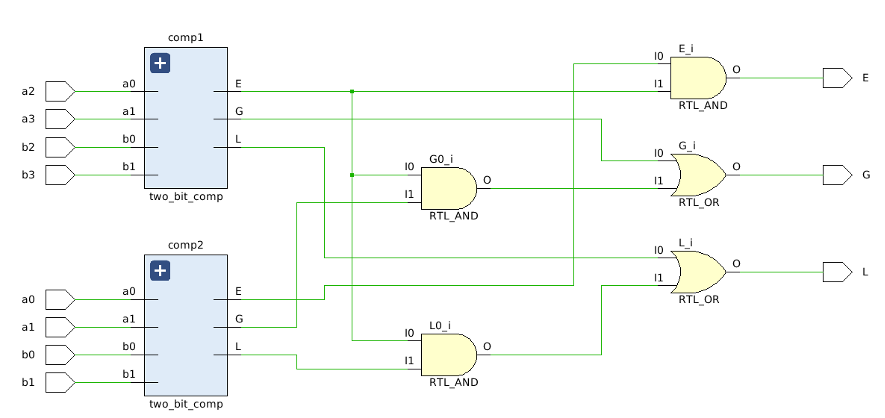As observed from the schematic, there are two 2-bit comparators and few gates to implement the 4-bit comparator in Verilog. In addition, the constraint file for the 4-bit comparator can be found on Github designed by Digilent.

## Simulation

The objective of the post is to compare the simulated waveform and actual observation on the Nexys board, so the waveforms can be randomly generated by using the random function in the testbench.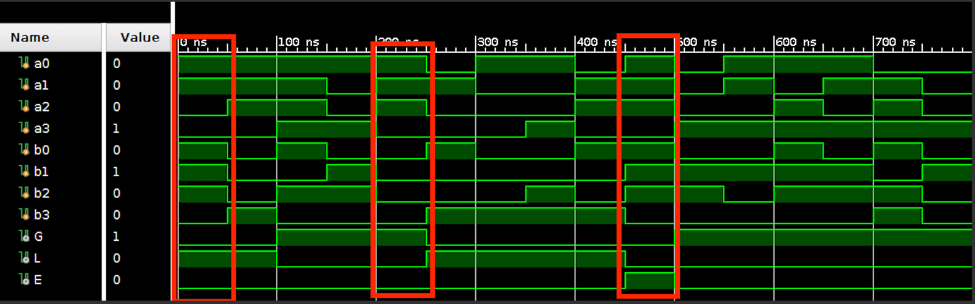## Final Result on Nexys Board

This time, I tested the part surrounded by red with Nexus board. Comparing the behavioral simulation with the actual results observed as LED output, it can be seen that it is working properly.